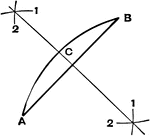### Bisect A Line

Method to draw the bisector of a line### Circle with Arc, Chord, Inscribed Angle, Circumscribed Polygon

Illustrations of a circle with arc, chord, inscribed angle, and circumscribed about a polygon.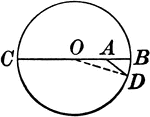### Circle With Diameter, Radius, Segment

Illustration showing a circle with a diameter, radius, and segment drawn.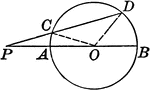### Circle With Diameter, Radius, Segment, Line

Illustration showing a circle with a diameter, radius, lines, triangle, and segment drawn.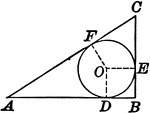### Circle Inscribed in a Right Triangle

Illustration showing the diameter of a circle inscribed in a right triangle is equal to the difference…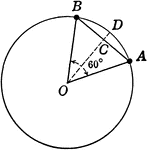### Circle With Sector and Segment labeled

Illustration of circle with sector and segment used to find area.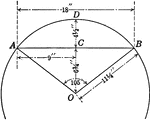### Circle With Sector and Segment labeled

Illustration of partial circle with sector and segment used to find area.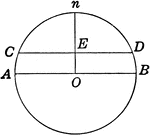### Circle With Diameter and Segment labeled

Illustration of circle with diameter and segment used to find area.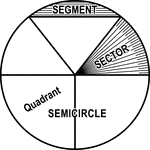### Circle with Segment, Semicircle, Sector, Quadrant, and Central Angle

Illustrations of a circle with segment, semicircle, sector, quadrant, and central angles drawn.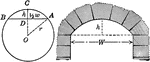### Segments of Circle and Arch

Illustration of circle with segments labeled and arch.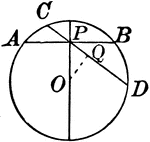### Circle With Various Chords

Illustration showing a circle with various chords drawn.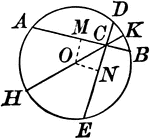### Circle With Various Chords

Illustration showing a circle with various chords drawn.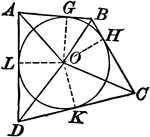### Circle With Various Chords And Tangents

Illustration showing a circle with various chords and tangents drawn.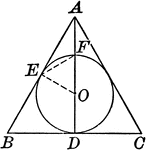### Circle With Various Chords And Tangents

Illustration showing a circle with various chords and tangents drawn.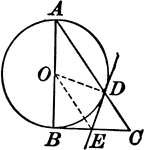### Circle With a Right Triangle

Illustration where one leg of a right triangle is the diameter of a circle. The tangent at the point…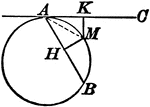### Circle With a Tangent Line and Chord

Illustration showing that from any point in the circumference of a circle, a chord and a tangent are…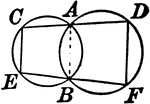### Two Intersecting Circles With Lines

Illustration showing 2 intersecting circles with a line drawn through each point of intersection terminated…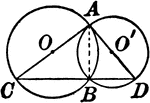### Two Intersecting Circles With Lines

Illustration showing 2 intersecting circles with a lines drawn that form a triangle.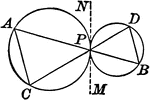### Tangent Circles With Chords

Illustration showing 2 circles with that touch each other and two lines drawn through the point of contact…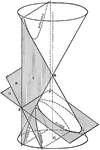### Conic Sections 3D

Three dimensional representation of the intersecting planes required to create the conic sections hyperbola,…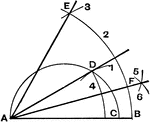### Construct Equilateral Triangle

Method to construct an equilateral triangle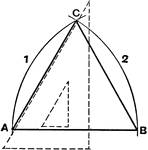### Construct Isosceles Triangle

Method to construct an isosceles triangle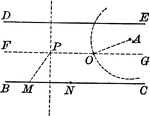### Construction of a Circle Through a Given Point that Cuts Chords of Given Lengths From Parallels

Illustration used to construct a circle that shall pass through a given point and cut chords of a given…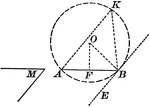### Construction to Describe a Segment of a Circle in Which an Angle Can Be Inscribed

Illustration of the construction used upon a given straight line, to describe a segment of a circle…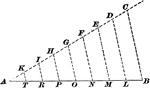### Construction Of Dividing A Line

Illustration used to show how to divide a given straight line into required number of equal parts.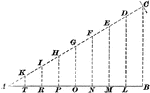### Construction Of Dividing A Line

Illustration used to show how to divide a given straight line into required number of equal parts.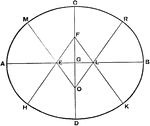### Ellipse Fifth Method

Draftsman's fifth method for drawing an ellipse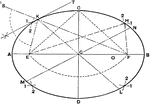### Ellipse First Method

Draftsman's first method for creating an ellipse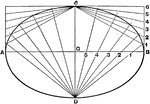### Ellipse Fourth Method Case 1

Draftsman's fourth method for drawing an ellipse, case 1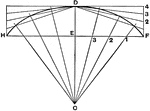### Ellipse Fourth Method Case 2

Draftsman's fourth method for drawing an ellipse, case 2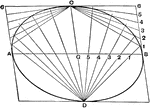### Ellipse Fourth Method Case 3

Draftsman's fourth method for drawing an ellipse, case 3### Ellipse Second Method

Draftsman's second method for drawing an ellipse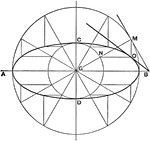### Ellipse Third Method

Draftsman's third method for drawing an ellipse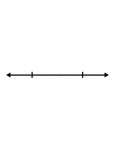### Flashcard of a Line Segment

A flashcard featuring an illustration of a Line Segment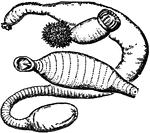### Gephyrea

Gepyrea is a name formerly given to a class of worms which included three families: (1) Priapulidae;…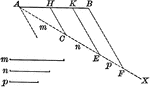### Line Divided Into Parts

Line divided into proportional parts.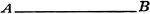### Straight Line

An illustration of a straight line/segment.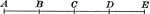### Straight Line With Points Divided Into Equal Parts

An illustration of a straight line with 5 points dividing it into 4 equal parts. Multiple of a given…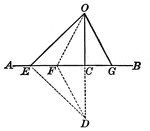### Lines Drawn From the Same Point in a Perpendicular to a Given line, Cutting Off Segments

Illustration showing two straight lines drawn from the same point in a perpendicular to a given line,…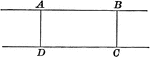### Parallel Lines With Equal Segments Between

Illustration of two parallel lines with equal segments between them. Two parallel lines are everywhere…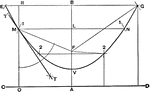### Parabola First Method

Draftsman's first method for drawing a parabola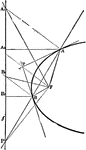### Parabola Foci Properties

The segments between the point of intersection of two tangents to a conic and their points of contact…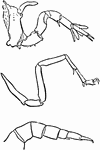### Prosomatic Limb

"Comparison of the sixth prosomatic limb of a recent scorpion (B), of Palaeophonus (C), and of Limulus…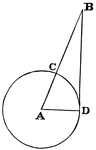### Circle with Secant

A secant is "a line which cuts a figure in any way. Specifically, in trigonometry, a line from the center…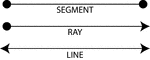### Definitions, Segment, Ray, Line

Illustrations of a segment, a ray, and a line that can be used when providing definitions.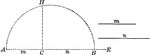### Semicircle

Semicircle with line drawn perpendicular to the diameter.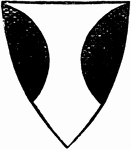### Flanch Shield

"The Flanch is formed of a segment of a circle placed on the side of the shield. It is always borne…### Sphere With 8-inch Diameter Cut by Planes

An 8-inch sphere cut by parallel planes, one 2 inches from center and the other 6 inches from center.…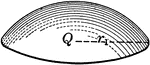### Zones or Segments of Spheres

An illustration of a zone of a sphere. A zone occurs when a sphere is cut by parallel planes that are…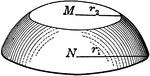### Zones or Segments of Spheres

An illustration of a zone of a sphere. A zone occurs when a sphere is cut by parallel planes that are…### Square With Diagonals and Lines Drawn

Illustration showing a square with diagonals, lines, and parallel lines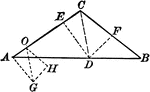### Triangle With Segments

Triangle with segments drawn.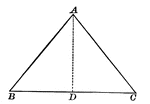### Isosceles Triangle With Interior Segment Drawn

Illustration showing an isosceles triangle with a segment inside. This can be used to show that in an…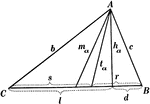### Parts Of A Triangle

Illustration used to show the various parts of a triangle: sides, angles, medians, altitudes, bisectors,…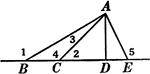### Segments and Angles in a Triangle

Illustration of a triangle with interior segments and angles labeled.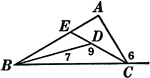### Segments and Angles in a Triangle

Illustration of a triangle with interior segments and angles labeled.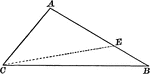### Triangle With Interior Segment Drawn

Illustration showing a triangle with a segment inside.